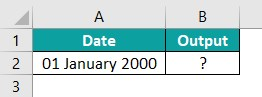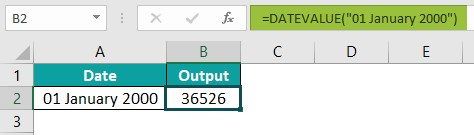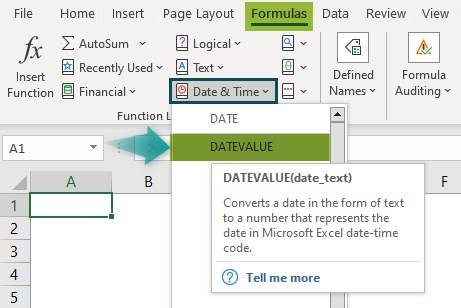# DATEVALUE In ExcelArticle byRoshan W## What Is DATEVALUE Function In Excel?

The DATEVALUE function in Excel returns the value of the date preset in Excel. The date must be entered as a text within double-quotes. This function is useful when the data is imported from files not supported by Excel, such as .pdf, .txt, or .csv. In such scenarios, the DATEVALUE function converts the dates into Excel recognizable format for further usage.

The Excel DATEVALUE function is an inbuilt “Date & Time” function, so we can insert the formula from the “Functional Library” or enter it directly in the worksheet.

For example, we will find the value of the given date using the DATEVALUE function.

Select cell B2, and enter the formula =DATEVALUE(“18-10-2022”).

The result is ‘44852’, as shown above.

###### Key Takeaways
• The DATE VALUE function converts any given date, in the Excel recognized format, into a serial number.
• The DATE VALUE function is used to convert text values containing both dates and times.
• The function has one mandatory argument, i.e., date_text, that has to be a valid date entered within double quotes.
• We can find the date values of the dates by using the DATE VALUE function along with the MID() and FIND() functions in Excel.
• We can perform arithmetic operations such as sum, product, difference, and division, if we have the start_date and the end_date, or with the results of the DATEVALUE calculations.

### DATEVALUE() Excel Formula

The syntax of the DATEVALUE Excel Formula is,

The argument of the DATEVALUE Excel Formula is,

• date_text: It is the text that represents the date in proper excel date format, which is entered in double quotes. It is a mandatory argument.

### How To Use Excel DATEVALUE function?

We can use the DATEVALUE function in 2 methods, namely,

1. Access from the Excel ribbon.
2. Enter in the worksheet manually.

#### Method #1 – Access from the Excel ribbon

1. Choose an empty cell for the output – select the “Formulas” tab – go to the “Function Library” – click the “Date & Time” option drop-down – select the “DATEVALUE” function, as shown below.

2. The “Function Arguments” window appears. Enter the argument value in the “Date_text” field – click “OK”, as shown below.

#### Method #2 – Enter in the worksheet manually

1. Select an empty cell for the output.
2. Type =DATEVALUE( in the selected cell. [Alternatively, type =D and double-click the DATEVALUE function from the list of suggestions shown by Excel.]
3. Enter the argument as cell values or cell references in Excel within double quotes.
4. Close the brackets, and press the “Enter” key.

We will calculate the date value using Excel DATEVALUE function.

In the table, the data is,

• Column A contains the Date.
• Column B displays the Output.

The procedure to evaluate the dates using the DATEVALUE function in Excel is,

First, enter the following formulas with the cell values,

• =DATEVALUE(“28-01-2010”) in cell B2.
• =DATEVALUE(“28-01-10”) in cell B3.
• =DATEVALUE(“28-Jan”) in cell B4.
• =DATEVALUE(“28-01-1900”) in cell B5.
• =DATEVALUE(“28-01-30”) in cell B6.
• Press “Enter” every time a formula is entered in a cell.

We get the above output, shown in column B. Column C is for our reference.

### Examples

We will understand some advanced scenarios with Excel DATEVALUE function examples.

#### Example #1

We will calculate the date value using the DATEVALUE function.

In the table, the data is,

• Column A contains the Date.
• Column B contains the Month.
• Column C contains the Year.
• Column D displays the Output.

The procedure to evaluate the dates using the DATEVALUE function in Excel is,

Select cell D2, enter the formula =DATEVALUE(A2&”/”&B2&”/”&C2), and press “Enter”.

The result is “44899”, as shown above.

#### Example #2

We will calculate the #VALUE! error using the Excel DATEVALUE function.

In the table, the data is,

• Column A contains the Date.
• Column B displays the Output.

The procedure to evaluate the dates using the DATEVALUE function is,

Select cell B2, enter the formula =DATEVALUE(A2), and press “Enter”.

The result is a “#VALUE!” error, as shown above because we entered the arguments cell reference without the double quotes.

#### Example #3

We will calculate the difference in date values using the DATEVALUE function.

In the table, the data is,

• Column A contains the DATEVALUE1.
• Column B contains the DATEVALUE2.
• Column C displays the Output of the difference in date values.

The steps to evaluate the dates by the DATEVALUE function in Excel are as follows:

Step 1: Select cell A2, enter the formula =DATEVALUE(“10-10-2022”), and press “Enter”. The result is 44844, as shown below.

Step 2: Next, select cell B2, enter the formula =DATEVALUE(“1-11-2020”), and press “Enter”. The result is 43841, as shown below.

Step 3: Select cell C2, enter the formula =A2-B2, i.e., =DATEVALUE(“10-10-2022”)-=DATEVALUE(“1-11-2020”), and press “Enter”.

The result is “708”, as shown above. Row 3 is for our reference.

#### Example #4

In the succeeding example that depicts the dates with the day, we will calculate the date value by extracting the date from the given data using the Excel DATEVALUE function with MID and FIND functions.

• The MID function in Excel is an Excel TEXT function that returns a specific number of characters from a text string, starting at the position specified, based on the number of characters specified.
• The FIND function in Excel is an Excel TEXT function that finds the location/position of an alphabet, character, or text string in the selected textual value.

In the table, the data is,

• Column A contains the Date.
• Column B displays the Output.

The procedure to evaluate the dates using DATEVALUE, MID and FIND is,

Select cell B2, enter the formula=DATEVALUE(MID(A2,FIND(” “,A2)+1,10)), and press “Enter”.

The result is “44853”, as shown above.

### Important Things to Note

• The function cannot convert a numeric value to a date. The entry must be a valid date in the right format.
• The #NUM! error occurs when the start_date exceeds the end_date.
• The #VALUE! error occurs when using the Excel DATEVALUE function on earlier dates before January 1, 1900, since this is the first date in Excel with the preset DATEVALUE as 1.

1. What does the DATEVALUE function do in Excel?

The DATEVALUE function converts a date entered as text to the date’s serial number as preset in Excel. When the data is imported from files not supported by Excel, such as .pdf, .txt, or .csv, the DATEVALUE function converts the dates into Excel recognizable format.

The syntax of the DATEVALUE function is =DATEVALUE(date_text)

2. How does the DATEVALUE function work in Excel?

The DATEVALUE function works in Excel by following the below steps;

1. Select an empty cell for the output.
2. Type =DATEVALUE( in the selected cell. [Alternatively, type =D and double-click the DATEVALUE function from the list of suggestions shown by Excel.]
3. Enter the argument as cell values or cell references within double quotes.
4. Close the brackets, and press the “Enter” key.

For example, the image depicts the date, and we will calculate the date value using the Excel DATEVALUE function.The procedure to evaluate the dates using DATEVALUE, MID and FIND is,

Select cell B2, enter the formula =DATEVALUE(“01 January 2000”), and press “Enter”.The result is “36526”, as shown above.

3. Where is the DATEVALUE function in Excel?

We can find and insert the DATEVALUE function in Excel as follows:
Choose an empty cell for the output – select the “Formulas” tab – go to the “Function Library” – click the “Date & Time” option drop-down – select the “DATEVALUE” function, as shown below.